index: click on a letter A B C D E F G H I J K L M N O P Q R S T U V W X Y Z A to Z index index: subject areas numbers & symbols sets, logic, proofs geometry algebra trigonometry advanced algebra & pre-calculus calculus advanced topics probability & statistics real world applications multimedia entrieswww.mathwords.com about mathwords website feedback

Integration by Parts

A formula used to integrate the product of two functions.

Formula: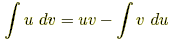Example 1: Evaluate.

Use u = x and dv = ex/2 dx. Then we get du = dx and v = 2ex/2. This can be summarized:

 u = x dv = ex/2 dx du = dx v = 2ex/2

It follows that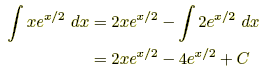Example 2: Evaluate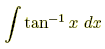.

Use the following:

 u = tan-1 x dv = dx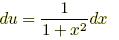v = x

Thus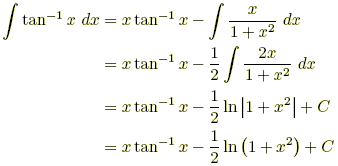Example 3: Evaluate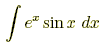.

Let I =. Proceed as follows:

 u = sin x dv = ex dx du = cos x dx v = ex

ThusNow use integration by parts on the remaining integral . Use the following assignments:

 u = cos x dv = ex dx du = –sin x dx v = ex

Thus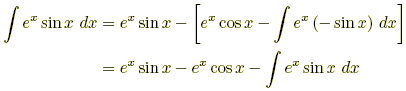Note thatappears on both sides of this equation. Replace it with I and then solve.We finally obtain